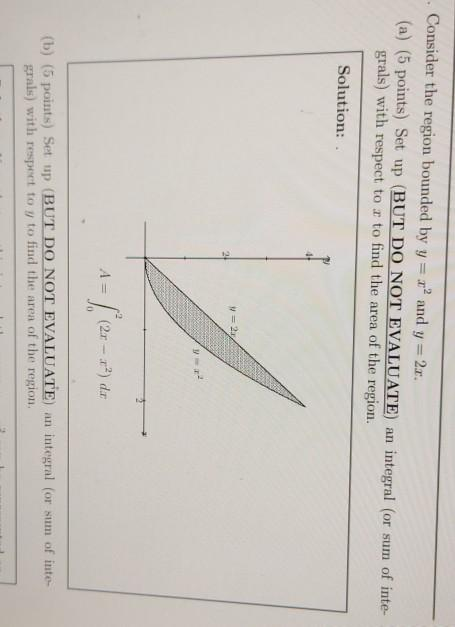### Create an Account

Already have account?

### Forgot Your Password ?

Home / Questions / . Consider the region bounded by y = x2 and y=2c. (a) (5 points) Set up (BUT DO NOT EVALUA...

# . Consider the region bounded by y = x2 and y=2c. (a) (5 points) Set up (BUT DO NOT EVALUATE) an integral (or sum of inte grals) with respect to r to find the area of the region. Solution: -1 (20-2²)

. Consider the region bounded by y = x2 and y=2c. (a) (5 points) Set up (BUT DO NOT EVALUATE) an integral (or sum of inte grals) with respect to r to find the area of the region. Solution: -1 (20-2²) 2) dor (b) (5 points) Set up (BUT DO NOT EVALUATE) an integral (or sum of inte grals) with respect to y to find the area of the region.Apr 14 2021 View more View Less

#### Answer (Solved)Subscribe To Get Solution